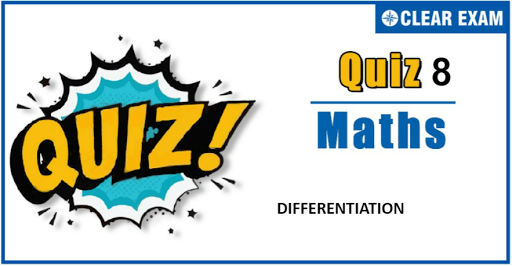## Differentiation Quiz

Important topics for Maths has been designed in such a way that it offers very practical and application-based learning to further make it easier for students to understand every concept or topic by correlating it with the day-to-day experiences.

Q1. If f''(x)=-f(x),where f(x)is a continuous double differentiable function and g(x)=f'(x).If F(x)=(f(x/2))^2+(g(x/2))^2 and F(5)=5 then F(10) is
•  0
•  5
•  10
•  25
Solution
(b) Given, f'' (x)=-f(x) ⇒g' (x)=-f(x) and f' (x)=g(x) …(i) Now, F(x)=(f(x/2))^2+(g(x/2))^2 ∴F'(x)=2(f(x/2)).f'(x/2).1/2 +2(g(x/2)).g'(x/2).1/2=0 [using Eq.(i)] ∴ F(x) is a constant ⇒ F(10)=F(5)=5

Q2.x=t cos⁡t,y=t+sin⁡t, then (d^2 x)/(dy^2) at t=π/2 is
•  (π+4)/2
•  -(π+4)/2
•  -2
•  None of these
Solution
(b) dx/dy=(dx/dt)/(dy/dt)=cos⁡t-t sin⁡t/(1+cos⁡t) ∴ (d^2 x)/(dy^2)=(d/dt (dx/dy))/(dy/dt) =(((-2 sin⁡t-t cos⁡t)(1+cos⁡t)-(cos⁡t-t sin⁡t)(-sin⁡t))/(1+cos⁡t)^2)/(1+cos⁡t) Now, put t=π/2

Q3.  Suppose the function f(x)-f(2x) has the derivative 5 at x=1 and derivative7 at x=2. The derivative of the function f(x)-f(4x) at x=1 has the value equal to
•   19
•  9
•  17
•  14
Solution
(a) y=f(x)-f(2x)⇒y'=f'(x)-2f'(2x) ⇒y'(1)=f'(1)-2f'(2)=5, and (1) y'(2)=f'(2)-2f'(4)=7 (2) Now let y=f(x)-f(4x) ⇒y'=f'(x)-4f'(4x) ⇒y'(1)=f'(1)-4f'(4) (3) Substituting the value of f'(2)=7+2f'(4) in(1), we get f'(1)-2(7+2f'(4))=5 f'(1)-4f'(4)=19

Q4. If y=(√(a+x)-√(a-x))/(√(a+x)+√(a-x)), then dy/dx is equal to
•  ay/x√(a^2-x^2)
•  ay/√(a^2-x^2 )
•  ay/(x√(x^2-a^2 ))
•  None of these
Q5. (d^20 y)/(dx^20 )(2 cos⁡x cos3x) is equal to
•  2^20 (cos⁡2x - 2^20cos⁡3x)
•  2^20 (cos⁡2x + 2^20cos⁡4x)
•  2^20 (sin⁡2x + 2^20sin⁡4x)
•  2^20 (sin⁡2x - 2^20sin⁡4x)
Solution
(b) y= 2cos⁡xcos⁡3x = cos⁡4x + cos⁡2x ⇒(d^20 y)/(dx^20)=4^20cos⁡4x + 2^20cos⁡2x

Q6. Let y=t^10+1 and x=t^8+1, then (d^2 y)/(dx^2 ) is
•  5/2 t
•  20t^8
• 5/(16t^6 )
•  None of these
Solution
(c) Here, y=t^10+1 and x=t^8+1 ∴t^8=x-1⇒t^2=(x-1)^(1/4) So, y=(x-1)^(5/4)+1 Differentiate both sides w.r.t. x, we get dy/dx=5/4(x-1)^(1/4) Again, differentiate both sides w.r.t., we get (d^2y)/(dx^2)=5/16(x-1)^(-3/4) ⇒(d^2y)/(dx^2)=5/(16(x-1)^(3/4))=5/(16(t^2 )^3)=5/(16t^6)

Q7.If f(x)=x^4 tan⁡(x^3)-xIn(1+x^2 ), then the value of (d^4 (f(x)))/(dx^4) at x=0 is
•  0
•  6
•  12
•  24
Q9.Let g(x) be the inverse of an invertible function f(x) which is differentiable at x=c, then g'(f(c)) equals
•  f'(c)
•  1/(f'(c))
•  f(c)
•  None of these
Solution
(b) Since g(x) is the inverse of function f(x), therefore gof(x)=I(x) for all x Now gof(x)=I(x),∀ x ⇒(gof)'(x)=1,∀ x ⇒g'(f(x))f'(x)=1,∀ x ⇒g'(f(x))=1/(f'(x)),∀ x ⇒g'(f(c))=1/(f'(c)) (putting x=c)

Q10. The derivative of y=(1-x)(2-x)…(n-x) at x=1 is
•  0
•  (-1)(n-1)!
•  n!-1
• (-1)^(n-1) (n-1)!
Solution
(b) dy/dx=-[(2-x)(3-x)…(n-x)+(1-x)(3-x)…(n-x)+⋯(1-x)(2-x)…(n-1-x)] At x=1 dy/dx=-[(n-1)!+0+⋯+0]=(-1)(n-1)!#### Written by: AUTHORNAME

AUTHORDESCRIPTION## Want to know more

Please fill in the details below:

## Latest NEET Articles\$type=three\$c=3\$author=hide\$comment=hide\$rm=hide\$date=hide\$snippet=hide

Name

ltr
item
BEST NEET COACHING CENTER | BEST IIT JEE COACHING INSTITUTE | BEST NEET & IIT JEE COACHING: Differentiation-Quiz-8
Differentiation-Quiz-8